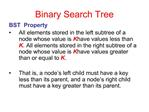DownloadDownload PresentationBinary Search Tree

# Binary Search Tree

Download Presentation## Binary Search Tree

- - - - - - - - - - - - - - - - - - - - - - - - - - - E N D - - - - - - - - - - - - - - - - - - - - - - - - - - -
##### Presentation Transcript

1. Binary Search Tree BST Property All elements stored in the left subtree of a node whose value is K have values less than K. All elements stored in the right subtree of a node whose value is K have values greater than or equal to K. That is, a node�s left child must have a key less than its parent, and a node�s right child must have a key greater than its parent.

2. Binary Search Tree

3. Binary Search Tree Operations on BST ADT Create a BST Insert an element Remove an element Find an element Clear (remove all elements) Display all elements in a sorted order

4. Binary Search Tree Inorder traversal Visit the left subtree, then the node, then the right subtree. Algorithm: If there is a left child visit it Visit the node itself If there is a right child visit it

5. Binary Search Tree Postorder traversal Visit each node after visiting its children. Algorithm: If there is a left child visit it If there is a right child visit it Visit the node itself

6. Binary Search Tree Preorder traversal Visit each node then visit its children. Algorithm: Visit the node itself If there is a left child visit it If there is a right child visit it

7. Binary Search Tree Insert Algorithm If value we want to insert < key of current node, we have to go to the left subtree Otherwise we have to go to the right subtree If the current node is empty (not existing) create a node with the value we are inserting and place it here.

8. Binary Search Tree For example, inserting �15� into the BST?

9. Binary Search Tree Delete Algorithm How do we delete a node form BST? Similar to the insert function, after deletion of a node, the property of the BST must be maintained.

10. Binary Search Tree There are 3 possible cases Node to be deleted has no children ? We just delete the node. Node to be deleted has only one child ? Replace the node with its child and make the parent of the deleted node to be a parent of the child of the deleted node Node to be deleted has two children

11. Binary Search Tree Node to be deleted has two children

12. Binary Search Tree Node to be deleted has two children Steps: Find minimum value of right subtree Delete minimum node of right subtree but keep its value Replace the value of the node to be deleted by the minimum value whose node was deleted earlier.

13. Binary Search Tree

14. Binary Search Tree template <class DataElem> class TreeNode { private: TreeNode *leftPtr; // pointer to left subtree DataElem data; // Node value TreeNode *rightPtr; // pointer to right subtree public: TreeNode( const DataElem &d ) { leftPtr = 0; data = d; rightPtr= 0; } DataElem getData() const { return data; } void setValue(DataElem val) { data = val; } };

15. Binary Search Tree class BSTree { private: TreeNode *rootPtr; // utility functions void insertNodeHelper (TreeNode **, const DataElem & ); void preOrderHelper ( TreeNode * ) const; void inOrderHelper ( TreeNode * ) const; void postOrderHelper ( TreeNode * ) const; void deleteNodeHelper (TreeNode *&, DataElem);

16. Binary Search Tree public: BSTree () { rootPtr = NULL; }; void insertNode ( const DataElem & ); void preOrderTraversal () const; void inOrderTraversal () const; void postOrderTraversal () const; void removeMin(); void deleteNode(DataElem); TreeNode* deleteMin(TreeNode *&); };

17. Binary Search Tree void Tree::insertNode( const DataElem &value ) { insertNodeHelper( &rootPtr, value ); } void Tree::deleteNode(DataElem val) { deleteNodeHelper( rootPtr, val ); }

18. Binary Search Tree // This function receives a POINTER to a pointer so the POINTER can be modified. void Tree::insertNodeHelper( TreeNode **ptr, const DataElem &value ) { if ( *ptr == NULL ) // tree is empty { *ptr = new TreeNode( value ); assert( *ptr != NULL ); } else // tree is not empty

19. Binary Search Tree // Else tree is not empty if ( value < ( *ptr )->data ) insertNodeHelper( &( (*ptr)->leftPtr ), value ); else if ( value > ( *ptr )->data ) insertNodeHelper( &( ( *ptr )->rightPtr ), value ); else cout << value << " is already in tree" << endl; }

20. Binary Search Tree void Tree::preOrderTraversal() const { preOrderHelper( rootPtr ); } void Tree::preOrderHelper(TreeNode *ptr ) const { if ( ptr != NULL ) { cout << ptr->data << ' '; preOrderHelper( ptr->leftPtr ); preOrderHelper( ptr->rightPtr ); } }